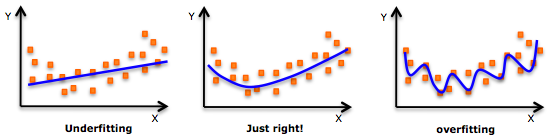# 10 Powerful Applications of Linear Algebra in Data Science (with Multiple Resour ...

If Data Science was Batman, Linear Algebra would be Robin. This faithful sidekick is often ignored. But in reality, it powers major areas of Data Science including the hot fields of Natural Language Processing and Computer Vision.I have personally seen a LOT of data science enthusiasts skip this subject because they find the math too difficult to understand. When the programming languages for data science offer a plethora of packages for working with data, people don’t bother much with linear algebra.That’s a mistake. Linear algebra is behind all the powerful machine learning algorithms we are so familiar with. It is a vital cog in a data scientists’ skillset. As we will soon see, you should consider linear algebra as a must-know subject in data science.And trust me, Linear Algebra really is all-pervasive! It will open up possibilities of working and manipulating data you would not have imagined before.In this article, I have explained in detail ten awesome applications of Linear Algebra in Data Science. I have broadly categorized the applications into four fields for your reference:I have also provided resources for each application so you can deep dive further into the one(s) which grabs your attention.Note: Before you read on, I recommend going through this superb article – Linear Algebra for Data Science. It’s not mandatory for understanding what we will cover here but it’s a valuable article for your budding skillset.I have come across this question way too many times. Why should you spend time learning Linear Algebra when you can simply import a package in Python and build your model? It’s a fair question. So, let me present my point of view regarding this.I consider Linear Algebra as one of the foundational blocks of Data Science. You cannot build a skyscraper without a strong foundation, can you? Think of this scenario:You want to reduce the dimensions of your data using Principal Component Analysis (PCA). How would you decide how many Principal Components to preserve if you did not know how it would affect your data? Clearly, you need to know the mechanics of the algorithm to make this decision.With an understanding of Linear Algebra, you will be able to develop a better intuition for machine learning and deep learning algorithms and not treat them as black boxes. This would allow you to choose proper hyperparameters and develop a better model.You would also be able to code algorithms from scratch and make your own variations to them as well. Isn’t this why we love data science in the first place? The ability to experiment and play around with our models? Consider linear algebra as the key to unlock a whole new world.The big question – where does linear algebra fit in machine learning? Let’s look at four applications you will all be quite familiar with.You must be quite familiar with how a model, say a Linear Regression model, fits a given data:But wait – how can you calculate how different your prediction is from the expected output? Loss Functions, of course.A loss function is an application of the Vector Norm in Linear Algebra. The norm of a vector can simply be its magnitude. There are many types of vector norms. I will quickly explain two of them:In this 2D space, you could reach the vector (3, 4) by traveling 3 units along the x-axis and then 4 units parallel to the y-axis (as shown). Or you could travel 4 units along the y-axis first and then 3 units parallel to the x-axis. In either case, you will travel a total of 7 units.This distance is calculated using the Pythagoras Theorem (I can see the old math concepts flickering on in your mind!). It is the square root of (3^2 + 4^2), which is equal to 5.But how is the norm used to find the difference between the predicted values and the expected values? Let’s say the predicted values are stored in a vector P and the expected values are stored in a vector E. Then P-E is the difference vector. And the norm of P-E is the total loss for the prediction.Regularization is a very important concept in data science. It’s a technique we use to prevent models from overfitting. Regularization is actually another application of the Norm.A model is said to overfit when it fits the training data too well. Such a model does not perform well with new data because it has learned even the noise in the training data. It will not be able to generalize on data that it has not seen before. The below illustration sums up this idea really well:Regularization penalizes overly complex models by adding the norm of the weight vector to the cost function. Since we want to minimize the cost function, we will need to minimize this norm. This causes unrequired components of the weight vector to reduce to zero and prev